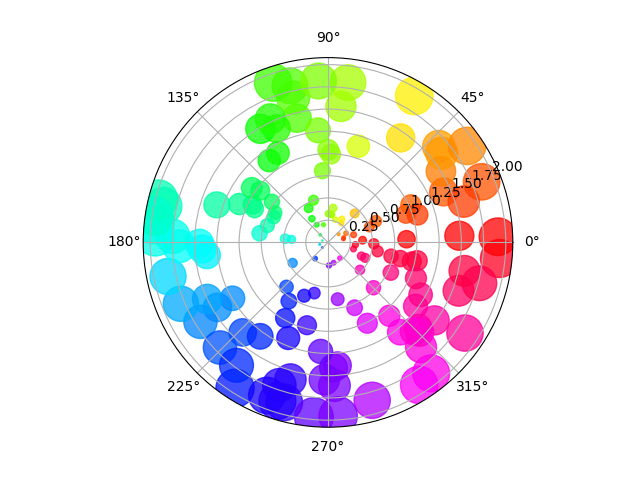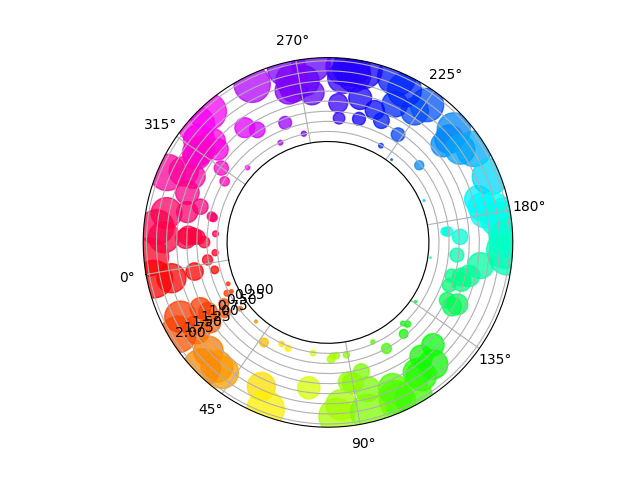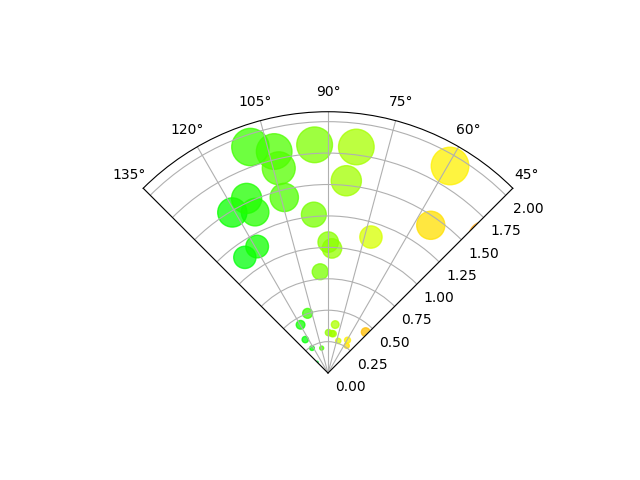# Scatter plot on polar axis#

Size increases radially in this example and color increases with angle (just to verify the symbols are being scattered correctly).

import numpy as np
import matplotlib.pyplot as plt

# Fixing random state for reproducibility
np.random.seed(19680801)

# Compute areas and colors
N = 150
r = 2 * np.random.rand(N)
theta = 2 * np.pi * np.random.rand(N)
area = 200 * r**2
colors = theta

fig = plt.figure()
c = ax.scatter(theta, r, c=colors, s=area, cmap='hsv', alpha=0.75)## Scatter plot on polar axis, with offset origin#

The main difference with the previous plot is the configuration of the origin radius, producing an annulus. Additionally, the theta zero location is set to rotate the plot.

fig = plt.figure()
c = ax.scatter(theta, r, c=colors, s=area, cmap='hsv', alpha=0.75)

ax.set_rorigin(-2.5)
ax.set_theta_zero_location('W', offset=10)## Scatter plot on polar axis confined to a sector#

The main difference with the previous plots is the configuration of the theta start and end limits, producing a sector instead of a full circle.

fig = plt.figure()
c = ax.scatter(theta, r, c=colors, s=area, cmap='hsv', alpha=0.75)

ax.set_thetamin(45)
ax.set_thetamax(135)

plt.show()References

The use of the following functions, methods, classes and modules is shown in this example:

Total running time of the script: ( 0 minutes 1.732 seconds)

Keywords: matplotlib code example, codex, python plot, pyplot Gallery generated by Sphinx-Gallery